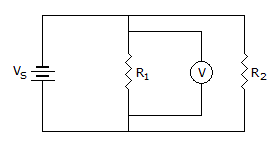# Electronics - Parallel Circuits - Discussion

### Discussion :: Parallel Circuits - Filling the Blanks (Q.No.5)

5.If the voltmeter indicates 12 V in the given circuit, the voltage across R2 equals ___.

 [A]. 12 V [B]. VS minus 12 V [C]. 0 V [D]. More information is needed to find the voltage across R2.

Explanation:

No answer description available for this question.

 Swathi Bhat said: (Feb 15, 2011) Since the 2 resistors are in parallel volage across them is the same= 12volts.

 Jai said: (Dec 4, 2013) Whenever any two resistor comes in parallel at the end of the circuit that time always the voltage at that parallel resistor is same as voltage at previous one parallel resistor.